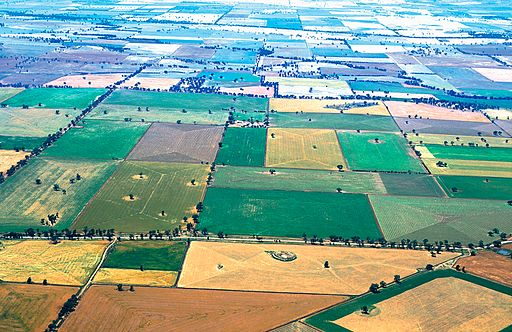# Reviewing The Greatest Common Factor

Student View (Opens in new window)## Instructor Overview

Students use a geometric model to investigate common factors and the greatest common factor of two numbers.

# Key Concepts

A geometric model can be used to investigate common factors. When congruent squares fit exactly along the edge of a rectangular grid, the side length of the square is a factor of the side length of the rectangular grid. The greatest common factor (GCF) is the largest square that fits exactly along both the length and the width of the rectangular grid. For example, given a 6-centimeter × 8-centimeter rectangular grid, four 2-centimeter squares will fit exactly along the length without any gaps or overlaps. So, 2 is a factor of 8. Three 2-centimeter squares will fit exactly along the width, so 2 is a factor of 6. Since the 2-centimeter square is the largest square that will fit along both the length and the width exactly, 2 is the greatest common factor of 6 and 8.

Common factors are all of the factors that are shared by two or more numbers.

The greatest common factor is the greatest number that is a factor shared by two or more numbers.

# Goals and Learning Objectives

• Use a geometric model to understand greatest common factor.
• Find the greatest common factor of two whole numbers equal to or less than 100.